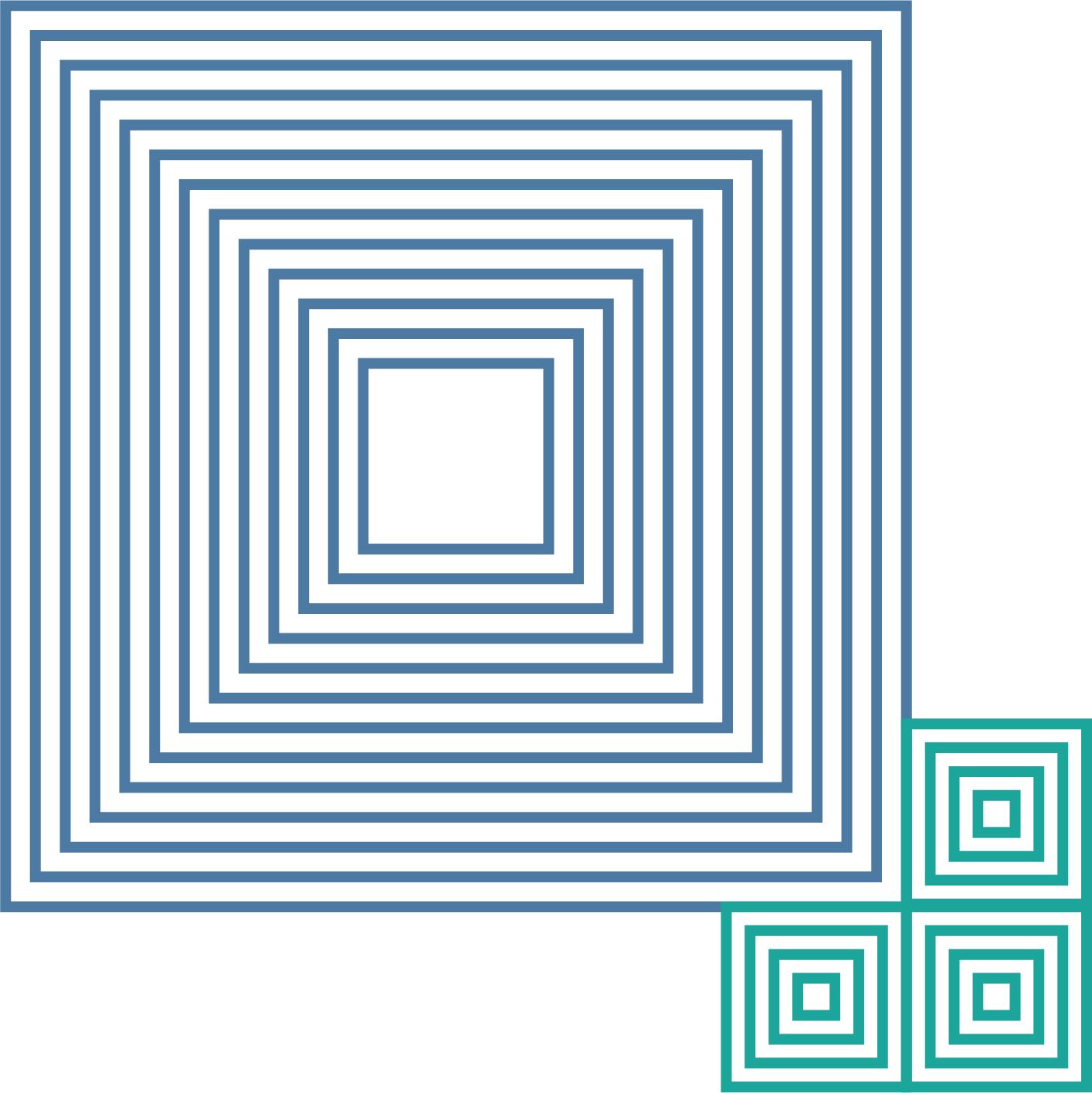QUANTUM COMPUTING THE SOFT WAY

# Quantum Logic Gates

From "Quantum Computing for the very curious" by Andy Matuschak and Michael Nielsen.

In Chapters 3 and 4 we learned about the state of a qubit. But in order to quantum compute, it’s not enough just to understand quantum states. We need to be able to do things with them! We do that using quantum logic gates.

A quantum logic gate is simply a way of manipulating quantum information, that is, the quantum state of a qubit or a collection of qubits. They’re analogous to the classical logic gates used in ordinary, everyday computers – gates such as the AND, OR, and NOT gates. And, much like classical gates’ role in conventional computers, quantum gates are the basic building blocks of quantum computation. They’re also a convenient way of describing many other quantum information processing tasks, such as quantum teleportation.

In this Chapter we’ll discuss several types of quantum logic gate. As we’ll see, the gates we discuss are sufficient to do any quantum computation. In particular, much as any classical computation can be built up using AND, OR, and NOT gates, the quantum gates we describe over the next few sections suffice to do any quantum computation.

Many of the quantum gates we’ll learn about are based on familiar classical logic gates. But a few are different. Those differences appear innocuous, almost trivial. But in those differences lies the power of quantum computation, and the possibility for quantum computers to be vastly superior to classical computers. Learning quantum gates is like learning basic vocabulary in a new language: there’s no getting round spending a fair bit of time working on it.

## The X gate

Let’s take a look at our very first quantum logic gate, the quantum NOT gate. As you can no doubt surmise, the quantum NOT gate is a generalization of the classical NOT gate. On the computational basis states the quantum NOT gate does just what you’d expect, mimicking the classical NOT gate. That is, it takes the $|0\rangle$ state to $|1\rangle$, and vice versa:

$NOT|0\rangle = |1\rangle$

$NOT|1\rangle = |0\rangle$

But the computational basis states aren’t the only states possible for a qubit. What happens when we apply the NOT gate to a general superposition state, that is, $\alpha|0\rangle+\beta|1\rangle$? In fact, it does pretty much the simplest possible thing: it acts linearly on the quantum state, interchanging the role of $|0\rangle$ and $|1\rangle$:

$NOT (\alpha|0\rangle +\beta|1\rangle) = \alpha|1\rangle+\beta |0\rangle$.

I’ve been using the notation NOT for the quantum NOT gate. But for historical reasons people working on quantum computing usually use a different notation, the notation X. And so the above may be rewritten:

$X (\alpha|0\rangle +\beta|1\rangle) = \alpha|1\rangle+\beta |0\rangle$.

I’ll use the terms X gate and NOT gate interchangeably.

Historically, the notation X traces its origin to 1927, when the physicist Wolfgang Pauli introduced an operation $s_x$​ (often written $\sigma_x$ in textbooks today) to help describe rotations of certain objects around the x spatial axis. This operation later became of interest to people working on quantum computing. But by that point the ss (and the connection to rotation) was irrelevant, and so $s_x$ just became X.

What we’ve seen so far are very algebraic ways of writing down the way the X gate works. There’s an alternate representation, the quantum circuit representation. In a quantum circuit we depict an X gate as follows: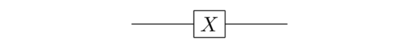The line from left to right is what’s called a quantum wire. A quantum wire represents a single qubit. The term “wire” and the way it’s drawn looks like the qubit is moving through space. But it's often helpful to instead think of left-to-right as representing the passage of time. So the initial segment of wire is just the passage of time, with nothing happening to the qubit. Then the X gate is applied. And then the quantum wire continues, leaving the desired output.

Sometimes we’ll put the input and output states explicitly in the quantum circuit, so we have something like: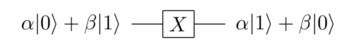So that’s the quantum circuit representation of the X gate. It is, in fact, our first quantum computation. A simple computation, involving just a single qubit and a single gate, but a genuine quantum computation nonetheless!

There’s a third representation for the X gate that’s worth knowing about, a representation as a $2 \times 2$ matrix:

$X = \left[ \begin{array}{cc} 0 & 1 \\ 1 & 0 \end{array} \right].$

To understand in what sense this is a representation of the NOT gate, recall that $\alpha|0\rangle + \beta|1\rangle$ is just the vector $\left[ \begin{array}{c} \alpha \\ \beta \end{array} \right]$. And so we have:

\begin{aligned} \left[ \begin{array}{cc} 0 & 1 \\ 1 & 0 \end{array} \right] (\alpha|0\rangle + \beta|1\rangle) & = \left[ \begin{array}{cc} 0 & 1 \\ 1 & 0 \end{array} \right] \left[ \begin{array}{c} \alpha \\ \beta \end{array} \right] \\ & = \left[ \begin{array}{c} \beta \\ \alpha \end{array} \right] \\ & = \alpha|1\rangle+\beta|0\rangle \\ & = X (\alpha|0\rangle + \beta|1\rangle). \end{aligned}

This tells us that X and $\left[ \begin{array}{cc} 0 & 1 \\ 1 & 0 \end{array} \right]$ act in exactly the same way on all vectors, and thus are the same operation. In fact, as we’ll see later, it turns out that all quantum gates can be thought of as matrices, with the matrix entries specifying the exact details of the gate.

By the way, regarding the X gate as a matrix clarifies what may have been a confusing point earlier. I wrote the X gate as having the action $X|0\rangle = |1\rangle, X |1\rangle = |0\rangle$. Implicitly – I never quite said this, though it’s true – the X gate is a mathematical function, taking input states to output states. But when we write functions we usually use parentheses, so why didn’t I write $X(|0\rangle)$ and similarly for $|1\rangle$? The reason is that for linear functions, i.e., matrices, it’s conventional to omit parentheses and just write $X|0\rangle$ – function application is just matrix multiplication.

A recap of what we just learned above can be found in this video of Michael Nielsen (perfect to watch while sitting on the bus!):

## Quantum wires

We’ve now seen a simple quantum circuit and quantum logic gate. But it’s not quite the simplest possible quantum circuit. The simplest possible quantum circuit does nothing at all. That is, it’s a single quantum wire: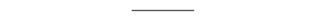This circuit is just a single qubit being preserved in time. To be more explicit, if some arbitrary quantum state $|\psi\rangle$ is input to the circuit, then the exact same state $|\psi\rangle$ is output (it’s common practice to use the Greek letter $\psi$ to denote an arbitrary quantum state):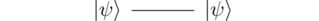Mathematically, this circuit is trivial. But physically it’s far from trivial. In many physical systems, the quantum wire is actually one of the hardest quantum computations to implement!

The reason is that quantum states are often incredibly fragile. If your qubit is being stored in some tiny system – perhaps a single photon or a single atom – then it’s very, very easy to disturb that state. It really doesn’t take much to upset an atom or a photon. And so while quantum wires are mathematically trivial, they can be one of the hardest elements to build in real systems.

That said, there are some systems where quantum wires are easy to implement. If you store your qubit in a neutrino then the state will actually be pretty well preserved. The reason is that neutrinos barely interact with other forms of matter at all – a neutrino can easily pass through a mile of lead without being disturbed. But while it’s intriguing that neutrinos are so stable, it doesn’t mean they make good qubits. The trouble is that since there’s no easy way of using ordinary matter to interact with them, we can’t manipulate their quantum state in a controlled fashion, and so can’t implement a quantum gate.

There’s a tension here that applies to many proposals to do quantum information processing, not just neutrinos. If we want to store the quantum state, then it’s helpful if our qubits only interact very weakly with other systems, so those systems don’t disrupt them. But if the qubits only interact weakly with other systems then that also makes it hard to manipulate the qubits. Thus, systems which make good quantum wires are often hard to build quantum gates for. Much of the art of designing quantum computers is about finding ways to navigate this tension. Often, that means trying to design systems which interact weakly most of the time, but some of the time can be caused to interact strongly, and so serve as part of a quantum gate.

## Quantum circuit

Let’s take a look at a simple multiple-gate quantum circuit. It’s just a circuit with two X gates in a row: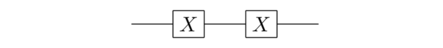It’s worth pausing for a moment, and trying to guess what this circuit does to the input state. It’s worth doing this even if you usually find this kind of guessing frustrating. Even when you get stuck, building up strategies for dealing with stuckness is part of learning any difficult subject. So take a minute or so now.

We’ll try two different ways of figuring out what’s going on. Here’s one approach, based on applying X twice to an arbitrary input state, $\alpha|0\rangle+\beta|1\rangle$. It’s simple algebra, using the fact X interchanges the $|0\rangle$ and $|1\rangle$ states:

\begin{aligned} X(X(\alpha|0\rangle+\beta|1\rangle)) & = X(\alpha|1\rangle + \beta|0\rangle) \\ & = \alpha|0\rangle + \beta|1\rangle \end{aligned}

So the net effect is to recover the original quantum state, no matter what that state was. In other words, this circuit is equivalent to a quantum wire: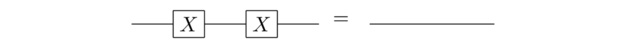A second way of seeing this is based on the matrix representation we found earlier for the X gate. Observe that if the input is some arbitrary quantum state $|\psi\rangle$, then after the first gate the state is $X|\psi\rangle$, and after the second gate the state is$X X |\psi\rangle$. Then we observe that the product X X is

\begin{aligned} XX & = \left[ \begin{array}{cc} 0 & 1 \\ 1 & 0 \end{array} \right] \left[ \begin{array}{cc} 0 & 1 \\ 1 & 0 \end{array} \right] \\ & = \left[ \begin{array}{cc} 1 & 0 \\ 0 & 1 \end{array} \right]. \end{aligned}.

That is, XX is the identity operation, and so the output $XX|\psi\rangle$ is just the original input $|\psi\rangle$. In other words, two XX gates is the same as a quantum wire. And so we’ve arrived at the same conclusion in a different way, using matrix multiplication. Doing such matrix multiplications is a pretty common way of analyzing quantum circuits.

## The Hadamard gate

We’ve seen our first quantum gate, the NOT or X gate. Of course, the X didn’t appear to do all that much beyond what is possible with a classical NOT gate. In this section I introduce a gate that clearly involves quantum effects, the Hadamard gate.

As with the X gate, we’ll start by explaining how the Hadamard gate acts on computational basis states. Denoting the gate by H, here’s what it does:

$H|0\rangle = \frac{|0\rangle +|1\rangle}{\sqrt 2}$

$H|1\rangle = \frac{|0\rangle -|1\rangle}{\sqrt 2}$

Of course, $|0\rangle$ and $|1\rangle$ aren’t the only quantum states. How does the Hadamard gate act on more general quantum states?

It won’t surprise you to learn that it acts linearly, as did the quantum NOT gate. In particular, the Hadamard gate takes a superposition $\alpha|0\rangle + \beta|1\rangle$ to the corresponding superposition of outputs:

$H(\alpha|0\rangle + \beta|1\rangle) = \alpha \left( \frac{|0\rangle+|1\rangle}{\sqrt 2} \right) + \beta \left( \frac{|0\rangle-|1\rangle}{\sqrt 2} \right).$

That’s a mess. We can make it less of a mess by combining the $|0\rangle$ terms together, and also the $|1\rangle$ terms together:

$H(\alpha|0\rangle + \beta|1\rangle) = \frac{\alpha+\beta}{\sqrt 2} |0\rangle + \frac{\alpha-\beta}{\sqrt 2}|1\rangle.$

That’s better, but still not pretty! Fortunately, we mostly won’t be dealing with such complex expressions. The only reason I’ve done it here is to be really explicit. Instead of dealing with such explicit expressions, we’ll mostly work with the H gate in its circuit and matrix representations (see below). Those let us focus at a more enlightening level of abstraction, rather than messing around with coefficients.

Indeed, much work on quantum computing is about attempting to develop ways of moving from low levels of abstraction to higher, more conceptual levels. Up to now most of our work has been at a very low level, seeming more an exercise in linear algebra than a discussion of a new model of computing. That perhaps seems strange. After all, if you were explaining classical computers to someone, you wouldn’t start in the weeds, with AND and NOT gates and the like. You’d start with a well-designed high-level programming language, and then bounce back and forth between different layers of abstraction. Modern computers aren’t just about logic gates – they’re at least as much about beautiful higher-level ideas: say, lazy evaluation, or higher-order functions, or homoiconicity, and so on.

I wish I could start with high-level abstractions for quantum computers. However, we’re still in the early days of quantum computing, and for the most part humanity hasn’t yet discovered such high-level abstractions. People are still scratching around, trying to find good ideas.

That’s an exciting situation: it means almost all the big breakthroughs are ahead. There’s a sense in which we still understand very little about quantum computing. That might sound surprising: after all, there are great big fat textbooks on the subject. But you could have written a great big fat textbook about the ENIAC computer in the late 1940s. It was, after all, a very complex system. That textbook would have looked intimidating, but it wouldn’t have been the final word in computing. For the most part the way we understand quantum computing today is at an ENIAC-like level, looking at the nuts-and-bolts of qubits and logic gates and linear algebra, and wondering what the higher-level understanding may be. The situation can be thought of as much like programming language design before the breakthroughs that led to languages such as Lisp and Haskell and Prolog and Smalltalk. That makes it a remarkable creative opportunity, a challenge for the decades and centuries ahead.

Speaking of nuts-and-bolts, let’s get back to the Hadamard gate. Here’s the circuit representation for the Hadamard gate. It looks just like the X gate in the circuit representation, except we change the gate label to H: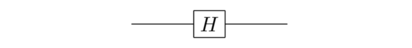Just like the X gate, H has a matrix representation:

$H = \frac{1}{\sqrt 2} \left[ \begin{array}{cc} 1 & 1 \\ 1 & -1 \end{array} \right]$.

To see that this matrix representation is correct, let’s check the action of the matrix on the $|0\rangle$ and the $|1\rangle$ states. Here we check for the $|0\rangle$ state:

\begin{aligned} \frac{1}{\sqrt 2} \left[ \begin{array}{cc} 1 & 1 \\ 1 & -1 \end{array} \right] |0\rangle & = \frac{1}{\sqrt 2} \left[ \begin{array}{cc} 1 & 1 \\ 1 & -1 \end{array} \right] \left[ \begin{array}{c} 1 \\ 0 \end{array} \right] \\ & = \frac{1}{\sqrt 2} \left[ \begin{array}{c} 1 \\ 1 \end{array} \right] \\ & = \frac{|0\rangle+|1\rangle}{\sqrt 2}. \end{aligned}​.​

That is, this matrix acts the same way as the Hadamard gate on the $|0\rangle$ state. Now let’s check on the $|1\rangle$ state:

\begin{aligned} \frac{1}{\sqrt 2} \left[ \begin{array}{cc} 1 & 1 \\ 1 & -1 \end{array} \right] |1\rangle & = \frac{1}{\sqrt 2} \left[ \begin{array}{cc} 1 & 1 \\ 1 & -1 \end{array} \right] \left[ \begin{array}{c} 0 \\ 1 \end{array} \right] \\ & = \frac{1}{\sqrt 2} \left[ \begin{array}{c} 1 \\ -1 \end{array} \right] \\ & = \frac{|0\rangle-|1\rangle}{\sqrt 2}. \end{aligned}​.​

So the matrix acts the same way as the Hadamard gate on both the $|0\rangle$ and $|1\rangle$ states. By the linearity of matrix multiplication it follows that the matrix acts the same way as the Hadamard on all input states, and so they are the same operation.

What makes the Hadamard gate interesting as a quantum gate? What can we use it to do?

We don’t (quite) have enough background to give precise answers to these questions yet. But there is an analogy which gives insight.

Imagine you are living in North Africa, thousands of years in the past, and decide for some reason that you want to get over to the Iberian peninsula. If you don’t yet have boats or some other reliable method of moving across large bodies of water, you need to go all the way across Africa, past the Arabian peninsula, up and around through Europe, back to the Iberian peninsula: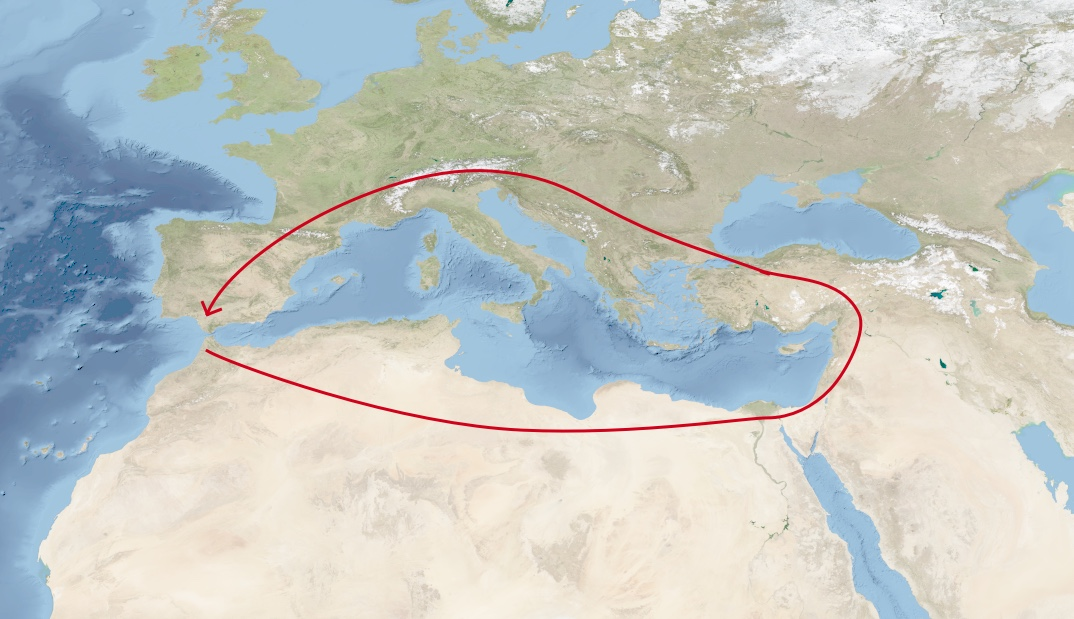Original photo source: Reto Stöckli, NASA Earth Observatory (2004)

Suppose, however, that you invent a new device, the boat, which expands the range of locations you can traverse. Then you can take a much more direct route over to the Iberian peninsula, greatly cutting down the time required: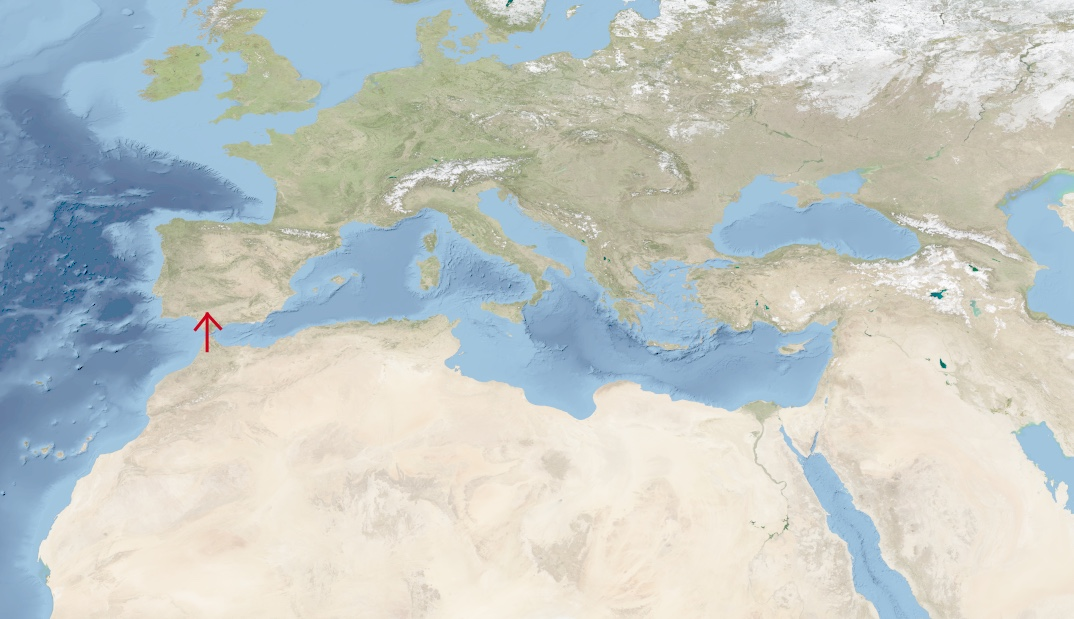What the Hadamard and similar gates do is expand the range of operations that it’s possible for a computer to perform. That expansion makes it possible for the computer to take shortcuts, as the computer “moves” in a way that’s not possible in a conventional classical computer. And, we hope, that may enable us to solve some computational problems faster.

Another helpful analogy is to the game of chess. Imagine you’re playing chess and the rules are changed in your favor, enabling your rook an expanded range of moves. That extra flexibility might enable you to achieve checkmate much faster because you can get to new positions much more quickly.

A similar thing is going on with the Hadamard gate. By expanding the range of states we can access (or, more precisely, the range of dynamical operations we can generate) beyond what’s possible on a classical computer, it becomes possible to take shortcuts in our computation.

We’ll see explicit examples in subsequent essays.

To get more familiar with the Hadamard gate, let’s analyze a simple circuit: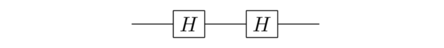What’s this circuit do?

Before we compute, it’s worth pausing for a second to try guessing the result. The point of guessing isn’t to get it right – rather, it’s to challenge yourself to start coming up with heuristic mental models for thinking about what’s going on in quantum circuits. Those mental models likely won’t be very good at first, but that’s okay – if you keep doing this, they’ll get better.

Here’s one heuristic you can use to think about this circuit: you can think of HH as sort of mixing the $|0\rangle$ and $|1\rangle$ states together. So if you apply HH twice to $|0\rangle$, perhaps it would thoroughly mix the $|0\rangle$ and $|1\rangle$ up together. What sort of state do you think would result, according to this heuristic? Do you believe the result? Why or why not? Can you think of other heuristics that might help you guess an answer?

Alright, let’s compute what actually happens. After we apply the first Hadamard to $|0\rangle$ we get

$\frac{|0\rangle+|1\rangle}{\sqrt{2}}​.$

Then we apply a second Hadamard gate. This takes the $|0\rangle$ term above to $\frac{|0\rangle+|1\rangle}{\sqrt 2}$ and the $|1\rangle$ term to $\frac{|0\rangle-|1\rangle}{\sqrt 2}$​, so the output is:

$\frac{1}{\sqrt 2} \left( \frac{|0\rangle+|1\rangle}{\sqrt 2} + \frac{|0\rangle-|1\rangle}{\sqrt 2} \right).$

If you look at the expression above, you’ll notice that the $|1\rangle$ terms cancel each other out, so you’re just left with the $|0\rangle$terms. Collecting them up, we’re left with the $|0\rangle$ state, same as we started with:

$\frac{1}{\sqrt 2} \left(\frac{|0\rangle}{\sqrt 2}+ \frac{|0\rangle}{\sqrt 2} \right) = |0\rangle.$

In a similar fashion, after we run the $|1\rangle$ state through the first Hadamard gate, we get:

$\frac{|0\rangle-|1\rangle}{\sqrt{2}}​.$

Then we apply a second Hadamard gate to get:

$\frac{1}{\sqrt 2} \left( \frac{|0\rangle+|1\rangle}{\sqrt 2} - \frac{|0\rangle-|1\rangle}{\sqrt 2} \right).$

This time it’s the $|0\rangle$ terms which cancel out, and the $|1\rangle$ terms reinforce. When we collect up these terms, we see that the output is just the $|1\rangle$ state, same as we started with. And so both the $|0\rangle$ and $|1\rangle$ states are left unchanged by this quantum circuit, and the net effect of the circuit is exactly the same as a quantum wire: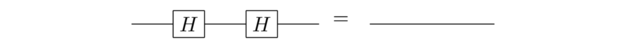There’s an alternate way of seeing this, which I’ll sketch out without working through in detail. It’s to note that if we input an arbitrary quantum state $|\psi\rangle$ to the circuit, then the output must be $H H |\psi\rangle$, i.e., the result of applying two HH matrices to $|\psi\rangle$. But if you just compute the matrix product HH it turns out to be the $2 \times 2$ identity matrix, $H H = I$. And so the output from the circuit must be the same as the input, $HH|\psi\rangle = I |\psi\rangle = |\psi\rangle$, just as from a quantum wire.

Of course, this result violates our intuitive guess, which was that two Hadamards would thoroughly mix up the $|0\rangle$ and the $|1\rangle$. It’s interesting to ponder what went wrong with our intuition, say by looking through the calculation for H acting twice on $|0\rangle$. You see that after the second gate the $|1\rangle$ terms exactly cancel one another out, while the $|0\rangle$ terms reinforce one another.

This seems innocuous, almost like a mathematical accident. Still, I draw your attention to it because this type of cancellation or reinforcement is crucial in many algorithms for quantum computers. Without getting into details, the rough way many such algorithms work is to first use Hadamard gates to “spread out” in quantum states like $\frac{|0\rangle+|1\rangle}{\sqrt 2}$ (or many-qubit analogs), i.e., in superpositions of multiple computational basis states. At the end of the algorithm they use clever patterns of cancellation and reinforcement to bring things back together again into one (or possibly a few, in the many-qubit case) computational basis state, containing the desired answer. That’s a somewhat vague and perhaps tantalizing description, but the point to take away is that the kind of cancellation-or-reinforcement we saw above is actually crucial in many quantum computations.

Once again, if you want to have a quick video recap of this section on Hadamard gates, here is your chance to listen to Michael Nielsen's in his wonderful Australian accent!

A different take on the introduction of Hadamard gate and phase gate can be learned by Prof. Artur Eckert in this video in which he explains single qubit interference and introduces the phase gate:

I'll conclude this section with a few simple exercises related to the Hadamard gate. They are given as additional challenges, they will help you gain insight into how this quantum gates work and, more in general, into the mathematics of quantum gates. Try them out!

Exercise: Verify that $HH = I$, where I is the $2 \times 2$ identity matrix, $I = \left[ \begin{array}{cc} 1 & 0 \\ 0 & 1 \end{array} \right]$.

Exercise: Suppose that instead of H we’d defined a matrix J by:

$J := \frac{1}{\sqrt 2} \left[ \begin{array}{cc} 1 & 1 \\ 1 & 1 \end{array} \right]$

At first, it might seem that $J$ would make an interesting quantum gate, along lines similar to H. For instance, $J|0\rangle = \frac{|0\rangle+|1\rangle}{\sqrt 2}​$, and $J|1\rangle = \frac{|0\rangle+|1\rangle}{\sqrt 2}$​. These are both good, normalized quantum states. But what happens if we apply J to the quantum state $\frac{|0\rangle-|1\rangle}{\sqrt 2}$​? Why does this make J unsuitable for use as a quantum gate?

Exercise: Consider the quantum circuit: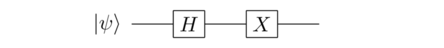Explain why the output from this circuit is $XH|\psi\rangle$, not $HX|\psi\rangle$, as you might naively assume if you wrote down gates in the order they occur in the circuit. This is a common gotcha to be aware of – it occurs because quantum gates compose left-to-right in the circuit representation, while matrix multiplications compose right-to-left.

## General single-qubit gates

So far we’ve learned about two quantum gates, the NOT and the Hadamard gate, and also about the measurement process that can be used to extract classical information from our quantum circuits. In this section, I return to quantum gates, and take a look at the most general single-qubit gate. To do that it helps to recall the matrix representations of the NOT and Hadamard gates:

\begin{aligned} X = \left[ \begin{array}{cc} 0 & 1 \\ 1 & 0 \end{array} \right]; \,\,\,\, H = \frac{1}{\sqrt 2} \left[ \begin{array}{cc} 1 & 1 \\ 1 & -1 \end{array} \right] \end{aligned}

If the input to these gates is the quantum state $|\psi\rangle$, then the output is $X|\psi\rangle$ and $H|\psi\rangle$, respectively.

A general single-qubit gate works similarly. In particular, a general single-qubit gate can be represented as a $2 \times 2$ unitary matrix, U. (If you’re rusty, I’ll remind you what it means for a matrix to be unitary in a moment, and you can just think of it as a matrix for now.) If the input to the gate is the state $|\psi\rangle$ then the output from the gate is $U|\psi\rangle$. And so the NOT and Hadamard gates correspond to the special cases where $U = X$ and $U = H$, respectively.

What does it mean for a matrix U to be unitary? It’s easiest to answer this question algebraically, where it simply means that $U^\dagger U = I$, that is, the adjoint of U, denoted $U^\dagger$, times U, is equal to the identity matrix. That adjoint is, recall, the complex transpose of U:

$U^\dagger := (U^T)^*$.

So for a $2 \times 2$ matrix, the adjoint operation is just:

$\left[ \begin{array}{cc} a & b \\ c & d \end{array} \right]^\dagger = \left[ \begin{array}{cc} a^* & c^* \\ b^* & d^* \end{array} \right].$.

(Note that the $\dagger$ is also sometimes called the dagger operation, or Hermitian conjugation, or just the conjugation operation. We’ll use all three terms on occasion.)

There are a few basic questions you might ask: why are single-qubit gates described by unitary matrices? And how can we get an intuitive feel for what it means for a matrix to be unitary, anyway? While the equation $U^\dagger U = I$ is easy to check algebraically, we’d like some intuition for what that equation means.

Another natural question is whether the NOT gate and the Hadamard gate are unitary? Of course, we’ll see that they are – I wouldn’t have described them as quantum gates if not – but we should go to the trouble of checking.

Yet another good question is whether there are useful examples of single-qubit gates that aren’t the NOT or Hadamard gates? The equation $U^\dagger U = I$ is all very well, but it’d be nice to have more concrete examples than just an abstract equation.

We’ll answer all these questions over the next few sections.

Let’s start by checking the unitarity of the Hadamard gate. We start by computing the adjoint of H:

$H^\dagger = \left( \left( \frac{1}{\sqrt 2} \left[ \begin{array}{cc} 1 & 1 \\ 1 & -1 \end{array} \right]\right)^T\right)^*.$

Note that taking the transpose doesn’t change the matrix, since it is already symmetric. And taking the complex conjugate doesn’t change anything, since all the entries are real. So we have $H^\dagger = H$, and thus $H^\dagger H = HH$. But we saw earlier in the essay that $HH = I$. So HH is, indeed, unitary.

Exercise: Show that X is unitary.

Exercise: Show that the identity matrix I is unitary.

Exercise: Can you find an example of a $2 \times 2$ matrix that is unitary, and is not I, X, or H?

For flavor, let’s give a few more examples of single-qubit quantum gates. Earlier in the essay, I mentioned that the NOT gate X was introduced by the physicist Wolfgang Pauli in the early days of quantum mechanics. He introduced two other matrices, Y and Z, which are also useful quantum gates. The three gates, X, Y, and Z are known collectively as the Pauli matrices. The Y and Z gates will be useful extra tools in our toolkit of quantum gates; in terms of the earlier analogy they expand the repertoire of moves we have available to us. They’re crucial, for example, in protocols such as quantum teleportation and quantum error correction.

The Y gate is similar to the X gate, but instead of 1s on the off-diagonal, it has i and -i, so it takes $|0\rangle$ to $i|1\rangle$ and $|1\rangle$ to $-i|0\rangle$:

$Y := \left[ \begin{array}{cc} 0 & -i \\ i & 0 \end{array} \right]$.

The Z gate leaves $|0\rangle$ unchanged, and takes $|1\rangle$ to -$|1\rangle$:

$Z := \left[ \begin{array}{cc} 1 & 0 \\ 0 & -1 \end{array} \right].$

Exercise: Show that the Y and Z matrices are unitary, and so legitimate quantum gates.

Another good example of a quantum gate is a rotation, the kind of matrix you’ve most likely been seeing since high school. It’s just the ordinary rotation of the 2-dimensional plane by an angle $\theta$:

$\left[ \begin{array}{cc} \cos(\theta) & -\sin(\theta) \\ \sin(\theta) & \cos(\theta) \end{array} \right]$.

You can easily check that this is a unitary matrix, and so it’s valid quantum gate. And sometimes it’s a very useful quantum gate!

That mostly wraps up our little library of single-qubit gates. At this point we know almost everything needed for quantum computation. There are just two extra elements needed: extending our description of single qubits to multiple qubits, and describing a simple two-qubit quantum gate. We’ll get to those things shortly – not surprisingly, they look much like what we’ve already seen.

A very useful way of "seeing" quantum gates is in terms of their action in the Bloch sphere representation.

Artur Ekert explain this beautifully in the two videos below:

# Time to Play!

You are now ready to apply your knowledge to program a real quantum computer! Try it out by visiting the Apply Section of QPlayLearn.

Contact

Social

© QPlayLearn 2020